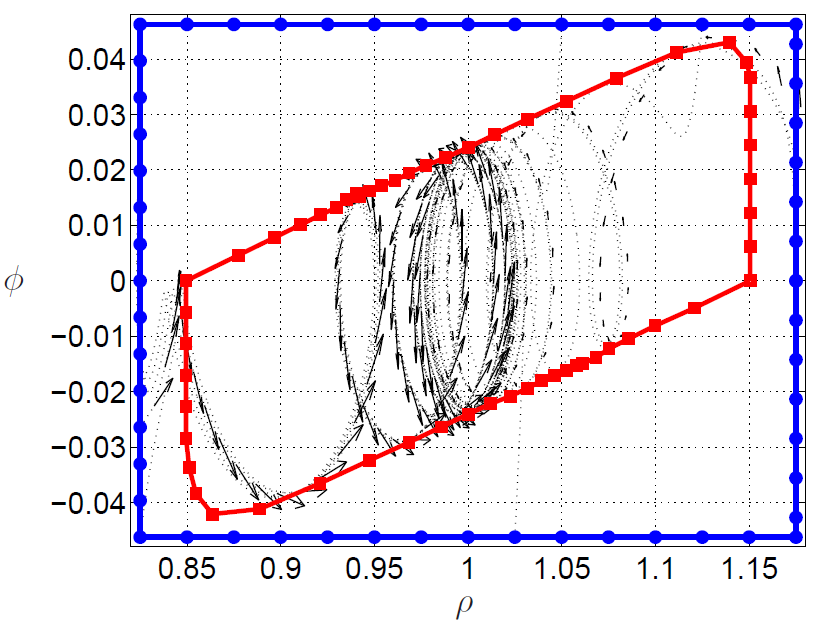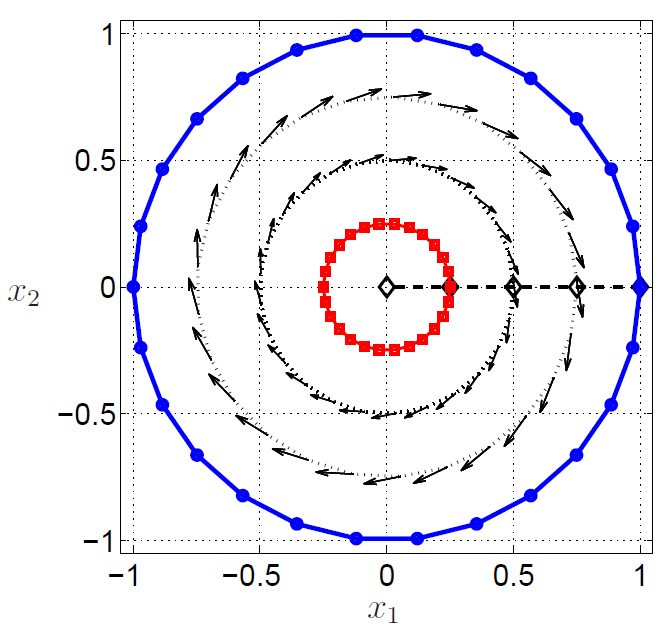# Robust Forward Invariant Sets for Nonlinear Systems (RFISs)

A dynamical system can be used to model a particular physical phenomenon of interest. It is common to have systems affected by additive perturbations. Explicit performance bounds, for such systems, are desirable. The concept of input-to-state stability (ISS) may sometimes provide a conservative performance bound for systems subjected to bounded time-varying disturbances. Compared to results based on ISS, it may be possible to establish more aggressive bounds on system performance by finding invariant sets. The shape and size of such an invariant set will depend on the properties of a system. This work focuses on developing a computational algorithm for finding invariant sets for systems subjected to bounded additive disturbances. These sets are named robust forward invariant sets (RFIS) as per . The authors in  provide analytical tools to construct robust forward invariant hexagons under bounded disturbances. Our goal is finding the smallest RFIS to obtain the tightest bounds on system performance. There exist many ways to construct invariant sets for dynamical systems. However, most methods existing in literature are analytical and valid for specific types of systems, or use numerical methods assuming some shape for an invariant set (enforced by the choice of a particular Lyapunov function). Minimizing the area occupied by sublevel sets of such a Lyapunov function, constrained by system dynamics, provides an estimate of a smallest invariant set. Since the shape is assumed apriori, it is not necessary that the solution obtained provides the smallest RFIS. Our work for computing an RFIS does not use Lyapunov functions or any particular optimization procedure. Newton-type methods for estimating a region of attraction are not used. Existing methods used to estimate the entire domain of attraction are not extended. A new approach is proposed to compute an RFIS for a perturbed system inspired by the notion of ‘Hamiltonians’. The idea  involves discretizing a domain of interest and starting with an initial guess of the boundary of the required invariant set. The proposed method iteratively evaluates points along proposed new sections of a possible boundary for the required set. During each evaluation, the angle between the vector field, and the tangent vector to a section of the possible boundary curve for the required set are compared. If the computed angle meets certain criteria, then the current point is stored as a possible choice for a boundary point. The choices for possible boundary points are updated every iteration. If the stored points satisfy certain termination criteria then the iterations stop and the required RFIS is found. Figures 1 and 2 show successful results obtained using the RFIS computation algorithm described here.Figure 1. Results of using the RFIS computation algorithm on a version of the curve tracking problemFigure 2. Results of using the RFIS computation algorithm with a simple system having circular trajectories

#### References

 M. Malisoff, F. Mazenc, and F. Zhang, “Stability and robustness analysis for curve tracking control using input-to-state stability,” IEEE Transactions on Automatic Control, vol. 57, no. 5, pp. 1320 – 1326, 2011.

 S. Mukhopadhyay, and F. Zhang “A path planning approach to compute the smallest robust forward invariant sets,” American Control Conference, pp. 1845 – 1850, 2014.

#### Said Al Abri wins Best Poster Award at CSL Student Conference